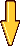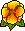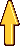## A quasidual function method for extracting edge stress intensity functions

Martin Costabel, Monique Dauge, Zohar YosibashWe present a new method for the computation of the coefficients of singularities along the edges of a polyhedron for second order elliptic boundary value problems. The class of problems considered includes:
  The heat transfer problem, in which case the edge coefficients can be qualified as Edge Flux Intensity Functions (EFIF),
  The linear three-dimensional elasticity, in which case the edge coefficients, the "Edge Stress Intensity Functions" (ESIF), represent the stress concentration along edges or crack fronts.

Our method uses an incomplete construction of 3D dual singular functions, which we call Quasidual Functions. They are based on explicitly known dual singular functions of 2D problems  Psi_0(x,y)  tensorized by test functions b(z)  along the edge, and combined with complementary terms  Psi_1(x,y) b'(z) + ...  improving their orthogonality properties with respect to the edge singularities.

Our method is aimed at the numerical computation of the stress intensity functions. It is suitable for a post-processing procedure in the finite element approximation of the solution of the boundary value problem. In a series of further works, we implement this method in order to compute EFIFs and ESIFs:

 In the paper presented, we implement the method for the Laplace operator and more general scalar second order operators.Accepted June 2003.

SIAM Journal on Mathematical Analysis Volume 35, Number 5 pp. 1177-1202 (2004)Pdf file (288 k)## Edge Flux Intensity Functions in Polyhedral Domains and their Extraction by a Quasidual Function Method

Netta Omer, Zohar Yosibash, Martin Costabel, Monique DaugeThe asymptotics of solutions to scalar second order elliptic boundary value problems in three-dimensional polyhedral domains in the vicinity of an edge involves:
(1)  A family of eigen-functions with their shadows,
(2)  The associated edge flux intensity functions (EFIFs), which are functions along the edges.

Utilizing the explicit structure of the solution in the vicinity of the edge we present a new method for the extraction of the EFIFs called Quasidual Function Method, whose mathematical foundation can be found in the paper. This method can be interpreted as an extension of the dual function contour integral method in 2-D domains, and involves the computation of a surface integral  J[R]  along a cylindrical surface of radius  R  away from the edge.

The surface integral  J[R]  utilizes special constructed extraction polynomials  b  together with the dual eigen-functions for extracting EFIFs. This accurate and efficient method provides a polynomial approximation of the EFIF along the edge whose order is adaptively increased so to approximate the exact EFIF. It is implemented as a post-solution operation in conjunction with the  p-version finite element method.

Numerical realization of some of the anticipated properties of the J[R]  integral are provided, and it is used for extracting EFIFs associated with different scalar elliptic equations in 3-D domains, including domains having edge and vertex singularities. The numerical examples demonstrate the efficiency, robustness and high accuracy of the proposed quasi-dual function method, hence its potential extension to elasticity problems.January 2004.

International Journal of Fracture 129 97-130, 2004.Pdf file (988 k)